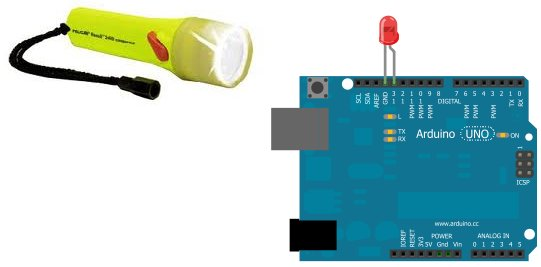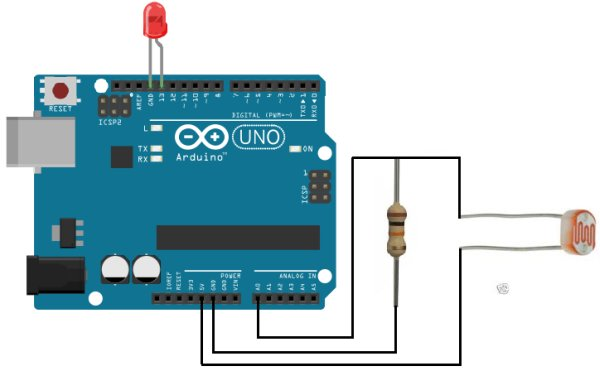# How to Build a Light Detector Circuit Using an Arduino

In this project, we will go over how to build a light detector circuit using an arduino.A light detector circuit is a circuit which can detect the presence or absence of light, depending on how we write our code to respond to the varying light levels. For example, if there is a great deal of light shining on our circuit, we can make anything that we want occur, such as have an LED light up. And we can do the same thing if there is a very low amount of light shining on our circuit. We could also just detect changes in light levels. We can detect a change when something passes in front of a light source, blocking the normal light to our circuit, etc.

For this circuit, we will make an LED light if there is a great amount of light hitting our circuit.

In another circuit, we will write a program for an LED to light when there is a very low amount of light, stimulating a night light.

For this project, the main component we will use is a photoresistor. When we place a photoresistor in series with a resistor, a voltage divider is formed. Voltage will be allocated to the 2 components based on the each one’s respective resistance. More voltage will fall across the component with the greater resistance, according to ohm’s law, V=IR. We will exploit this principle in this circuit to determine the light level that our circuit is exposed to.

### Components Needed for the Light Detector Circuit

• Photoresistor
• 10KΩ Resistor
• Arduino

### Light Detector Circuit Schematic

The schematic for the light detector circuit is shown below:### Code

The code to turn on an LED when sound is detected is shown below.

//these define the pin connections const int ledPin=13; //the code will flash the LED connected to digital pin 13
const int sensorPin= 0; //Sensor pin connects to analog pin A0

int level; //the variable that will hold the light level reading

const int threshold=800; //this represents the threshold voltage. If voltage is below 800, this triggers the LED to turn on

For more detail: How to Build a Light Detector Circuit Using an Arduino

#### This Post / Project can also be found using search terms:

• Light Detector Circuit
• light detector circuit arduino